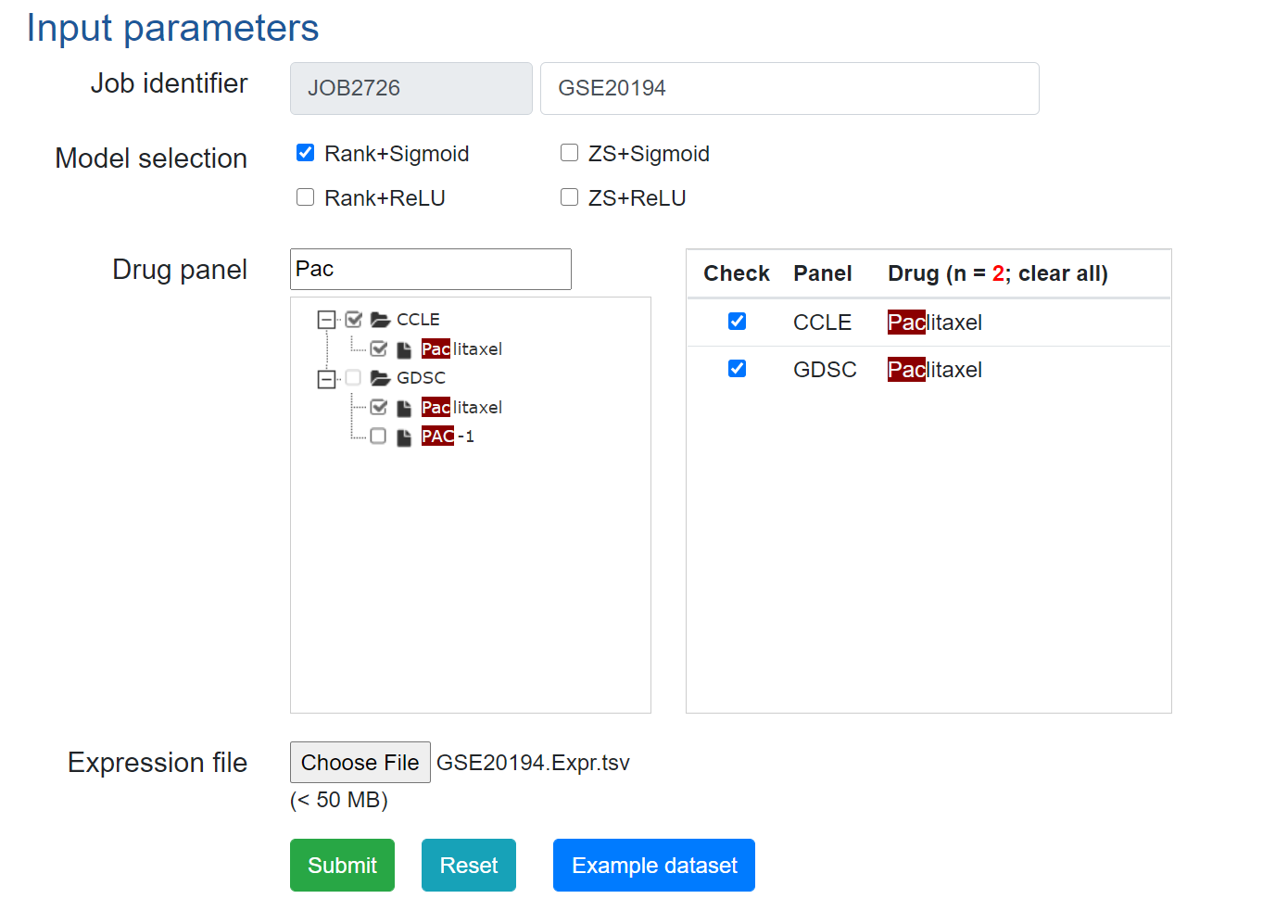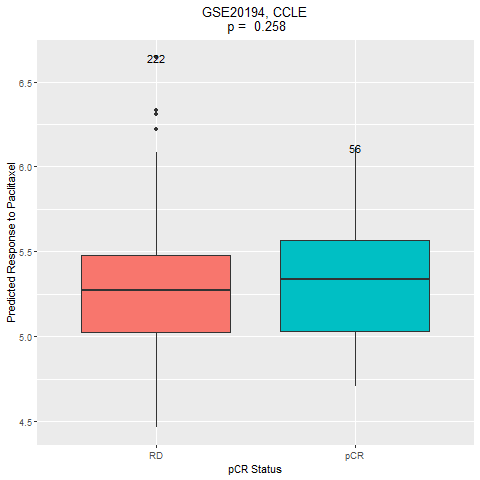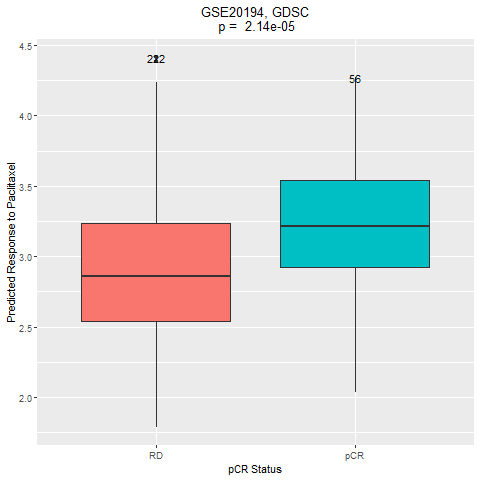## Run DrVAEN on dataset: GSE20194

#### 1. Dataset summary

The human breast cancer (BR) data set (endpoints D and E) was contributed by the University of Texas M. D. Anderson Cancer Center (MDACC, Houston, TX, USA). Gene expression data from 230 stage I-III breast cancers were generated from fine needle aspiration specimens of newly diagnosed breast cancers before any therapy. The biopsy specimens were collected sequentially during a prospective pharmacogenomic marker discovery study between 2000 and 2008. These specimens represent 70-90% pure neoplastic cells with minimal stromal contamination. Patients received 6 months of preoperative (neoadjuvant) chemotherapy including paclitaxel, 5-fluorouracil, cyclophosphamide and doxorubicin followed by surgical resection of the cancer. Response to preoperative chemotherapy was categorized as a pathological complete response (pCR = no residual invasive cancer in the breast or lymph nodes) or residual invasive cancer (RD), and used as endpoint D for prediction. Endpoint E is the clinical estrogen-receptor status as established by immunohistochemistry. RNA extraction and gene expression profiling were performed in multiple batches over time using Affymetrix U133A microarrays. Genomic analysis of a subset of this sequentially accrued patient population were reported previously. For each endpoint, the first 130 cases were used as a training set and the next 100 cases were used as an independent validation set.

#### 2. Get and prepare dataset

The dataset can be accessed using the code:

```  #setwd("/path/to/GSE20194")
library("GEOquery")
library("limma")
library("preprocessCore")

Sys.setlocale(category = "LC_ALL", locale = "us")

###################################################################################################
# code chunk: GSE20194
###################################################################################################

gse <- getGEO("GSE20194", GSEMatrix = TRUE)
exprs(gse[]) -> datExpr0

normalize.quantiles(datExpr0) -> datExprLQ
dimnames(datExprLQ) = dimnames(datExpr0)

gpl = getGEO("GPL96")
Table(gpl) -> anno

grep("/", anno[,11]) -> check
anno = anno[-check,]
anno = anno[anno[,11]!="", ]

datExprLQ = datExprLQ[which(rownames(datExprLQ) %in% anno[,1]),]
match(rownames(datExprLQ), anno[,1]) -> ii
symbol = anno[ii, 11]

apply(datExprLQ, 2, function(u)tapply(u, symbol, median)) -> expr.mat

write.table(format(expr.mat, digits=4),file="GSE20194.Expr.tsv", row.names=T, quote=F, sep="\t")

pData(gse[]) -> pheno.anno
save(expr.mat, pheno.anno, file="GSE20194.RData")

```

The genes will be mapped to our backend gene list that are used for drug response prediction. The full gene list is here

#### 3. Predict drug responses using DrVAEN.

Once the gene expression matrix file is genereted, we can upload the file to DrVAEN to get the predicted drug response data.Then download the predicted responses for further analysis.

#### 4. Further analysis: Compare the drug responses between the two groups (pCR vs RD).

Here we present the example codes for the analysis.

```library(glmnet)
load("GSE20194.RData")

## CCLE
drug_response = read.table("ranksigmoid_CCLE.pred.txt", as.is=T, header=T)

### all
dat = data.frame(cbind(Response = drug_response[, "Paclitaxel"], pCR=pheno.anno[,"pcr_vs_rd:ch1"]))
dat[,1] = as.numeric(as.character(dat[,1]))
t.test(dat[,1] ~ dat[,2])

##### plot
library("ggplot2")

give.n <- function(x){
return(c(y = max(x), label = length(x)))
}

### all
dat = data.frame(cbind(Response = drug_response[, "Paclitaxel"], pCR = pheno.anno[,"pcr_vs_rd:ch1"]))
dat[,1] = as.numeric(as.character(dat[,1]))
dat[,2] <- factor(dat[,2], levels = c("RD", "pCR"))

pvalue = t.test(dat[,1] ~ dat[,2])\$p.value

p5 = ggplot(dat, aes(x=pCR, y=Response, fill=pCR)) + geom_boxplot() +
labs(title=paste("GSE20194, CCLE\n", "p = ", format(pvalue, digits=3)), x="pCR Status", y = "Predicted Response to Paclitaxel") +
theme(legend.position = "none", plot.title = element_text(hjust=0.5)) +
stat_summary(fun.data = give.n, geom = "text")

png("CCLE.png")
print(p5)
dev.off()

## To analyse the predicted drug response data from GDSC model, please just change "CCLE" to "GDSC" in the above codes.
```